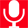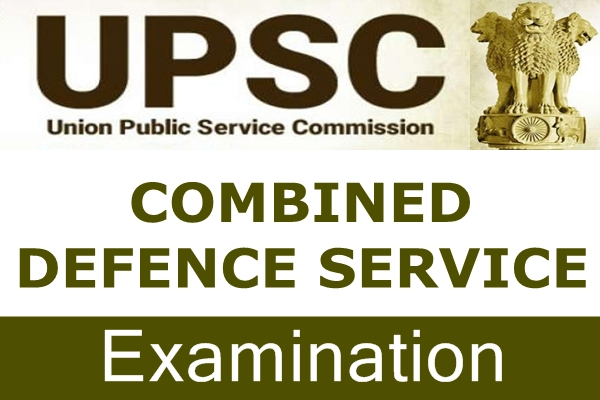Tuesday, July 5, 2022# Combined Defence Services (CDS) Examination: SyllabusIntroduction Eligibility Examination Application Procedure Syllabus Exam Date 2022

English

The question paper will be designed to test the candidates' understanding of English and workman-like use of words.

General Knowledge

General Knowledge, including knowledge of current events and such matters of everyday observation and experience in their scientific aspects as may be expected of an educated person who has not made a special study of any scientific subject. The paper will also include questions of History of India and Geography of a nature which candidates should be able to answer without special study.

Elementary Mathematics

Arithmetic:

Number system, Natural numbers, Integers, Rational and Real numbers. Fundamental Operations-addition, subtraction, multiplication, division, square roots, decimal fractions.

Unitary method:

Time and distance, time and work, percentages, applications to simple and compound interest, profit and loss, ratio and proportion, variation.

Elementary Number Theory:

Division algorithm, Prime and composite numbers, Tests of divisibility by 2, 3, 4, 5, 9 and 11, Multiples and factors, Factorization Theorem, H.C.F. and L.C.M.,

Algebra:

Basic operations, simple factors, Remainder Theorem, H.C.F., L.C.M., Theory of Polynomials, Solutions of quadratic equations, relation between its roots and coefficients (only real roots to be considered).

Simultaneous linear equations in two unknowns-analytical and graphical solutions. Simultaneous linear in-equations in two variables and their solutions. Practical problems leading to two simultaneous linear equations or in-equations in two variables or quadratic equations in one variable and their solutions.

Set language and set notation, Rational expressions and conditional identities, Laws of indices.

Trigonometry:

Sine x, Cosine x, Tangent x when 0° < x< 90°. Values of sin x, cos x and tan x, for x = 0°, 30°, 45°, 60° and 90°.
Simple trigonometric identities. Use of trigonometric tables.
Simple cases of heights and distances.

Geometry:

Lines and angles, Plane and plane figures.
Theorems on:

1. Properties of angles at a point
2. Parallel lines
3. Sides and angles of a triangle
4. Congruency of triangles
5. Similar triangles
6. Concurrence of medians and altitudes
7. Properties of angles, sides and diagonals of a parallelogram, rectangle and square
8. Circle and its properties, including tangents and normals
9. Loci.

Mensuration

Areas of squares, rectangles, parallelograms, triangle and circle. Areas of figures, which can be split up into the figures (Field Book). Surface area and volume of cuboids, lateral surface and volume of right circular cones and cylinders. Surface area and volume of spheres.

Statistics-Collection and tabulation of statistical data. Graphical representation-frequency polygons, histograms, bar charts, pie charts, etc. Measures of Central Tendency.

Find it Useful ? Help Others by Sharing Online